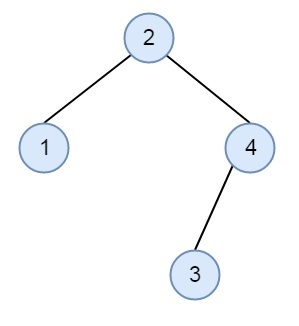# Check for Identical BSTs without building the trees in C++

We have two arrays to represent the elements of the BST. If we take elements from that array from left to right, and form the BST, then by taking from both the arrays, we will make the same BST. We have to check whether both are forming the same or not. But the constraint is we cannot make the BST. Suppose two arrays are {2, 4, 1, 3}, and {2, 1, 4, 3}, then if we see, both of these sequences can form same BST.The approach is simple. As we know, the elements of left subtree are smaller than root and the elements of the right subtree are greater than root. These two lists can represent same BST if for each element x, the elements in left and right subtrees of x appear after it in both arrays. Same is true for roots of left and right subtrees. We will check whether next smaller and greater elements are same in both arrays. This same property is checked recursively for left and right subtrees.

## Example

#include <iostream>
using namespace std;
bool isSameCheckHelper(int tree1[], int tree2[], int n, int i1, int i2, int min, int max) {
int j, k;
for (j = i1; j < n; j++)
if (tree1[j] > min && tree1[j] < max)
break;
for (k = i2; k < n; k++)
if (tree2[k] > min && tree2[k] < max)
break;
if (j==n && k==n) //If the parent element is leaf in both arrays
return true;
if (((j==n)^(k==n)) || tree1[j]!=tree2[k])
return false;
return isSameCheckHelper(tree1, tree2, n, j+1, k+1, tree1[j], max) &&
// for Right Subtree
isSameCheckHelper(tree1, tree2, n, j+1, k+1, min, tree1[j]); // for Left Subtree
}
bool areBSTSame(int first[], int second[], int n) {
return isSameCheckHelper(first, second, n, 0, 0, INT_MIN, INT_MAX);
}
int main() {
int first[] = {8, 3, 6, 1, 4, 7, 10, 14, 13};
int second[] = {8, 10, 14, 3, 6, 4, 1, 7, 13};
int n=sizeof(first)/sizeof(first);
if(areBSTSame(first, second, n)) {
cout << "Two BSTs are same";
} else {
cout << "Two BSTs are not same";
}
}

## Output

Two BSTs are same